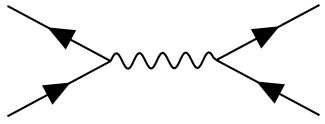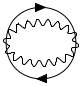/ tikz-feyn Public

A LaTeX package to draw Feynman diagrams using TikZ

# transientlunatic/tikz-feyn

Switch branches/tags
Nothing to show

A tag already exists with the provided branch name. Many Git commands accept both tag and branch names, so creating this branch may cause unexpected behavior. Are you sure you want to create this branch?

## Files

Failed to load latest commit information.
Type
Name
Commit time

# Tikz-feyn

Tikz-feyn is a LaTeX package which generates Feynman diagrams using the PGF/TikZ package.

Feature requests, bugs, feedback, and comments are always welcome!

The documentation for this package is very very lacking at the moment, and it's something I'll improve. I promise. When I find the time.

I originally created this package to make typing notes for a quantum field theory class easier (read I was procrastinating), but now it's there for the whole world to use,

# Requirements

You'll need the tikz, xparse, and ifthen packages for LaTeX, all of which are available in texlive.

# Usage

\usepackage{tikz-feyn}

A Feynman diagram can be constructed in one of two environments:

In the current implementation of Tikz-feyn each Feynman diagram is composed of a number of columns. For example,contains four columns. We construct a column using the \tfcol macro:

\tfcol(0,0){a, b, ..., z}[<vertical space>]

This creates a column of vertices, each equally-spaced, with the names, a, b, through to z. The bottom of the column will be aligned to the coordinates in the first, optional, argument.

To join vertices with edges use the \tf macro, for example

\tf[f]{a1,a2}

joins vertices a1 annd a2 with a fermion. The full set of arguments fot \tf is

\tf[<particle>]{<vertices>}[<inner direction>]

If you include a new vertex inside the list of vertices it will be created between the first and last vertices in the list, and its positioning can be dictated by the third argument as either u (up), d (down), l (left), or r (right). So a more complex example,

\tf[f]{a1,c1,a2}[r]

joins a1 to a2 with a fermion, but it creates a vertex called c1 in a column to the right of them, and vertically half-way between them.

To see all of this in action, let's have a full example of a diagram:

\begin{tfeyn}
\tfcol{a1, a2}
\tfcol{b1, b2}
\tf[f]{a1,c1,a2}[r]
\tf[f]{b1,c3,b2}[l]
\tf[p]{c1, c3}
\end{tfeyn}

So we set up two sets of vertices, a1 and a2, and b1, and b2. Next we connect a1 to a2 with a fermion, and we make a node, c1 half way along that edge, to the right of the a column, and we do something similar on the b column, but to the left. We can now connect c1 and c2 with a photon (p), and we have the completed diagram:It's possible to do all sorts of other fancy stuff, thanks to the underlying TikZ package.

Arcs and loops can be created within diagrams by adding the l style to a \tf macro:

\tf[p,l]{v4,v6}

and if necessary, it can be customised by adding a looseness key:

\tf[p,l, looseness=1]{i,o}

which adjusts the circularity of the arc. For an extreme example, take a diagram composed only loops and arcs:

\begin{tfeyn}
\tfcol{i}[1cm]
\tfcol{o}
\tf[p,l, looseness=1]{i,o}
\tf[p,l, looseness=1]{o,i}
\tf[f,l]{i,o}
\tf[f,l]{o,i}
\end{tfeyn}

which produces the outputAny TikZ style can be applied to any vertex, so to make a red photon just write

\tf[p,red] {i,o}

for example.

# Lit of Supplied Edges

Each particle in a Feynman diagram is represented as an edge, and in Tikz-feyn, each particle is created by styling an edge. The following styles are available:

Particle Style name(s) Example Image
Photon p, photon \tf[p]{i,o}
W Boson w, wboson \tf[w]{i,o}
Z Boson z, zboson \tf[z]{i,o}
Higgs Boson h, higgs \tf[h]{i,o}
Gluon g, gluon \tf[g]{i,o}
Graviton G, graviton \tf[G]{i,o}
Fermion f, fermion \tf[f]{i,o}

It should be fairly easy to implement your own style if you want to add some esoteric particle I don't know about!

A LaTeX package to draw Feynman diagrams using TikZ

## Releases

No releases published

## Packages 0

No packages published